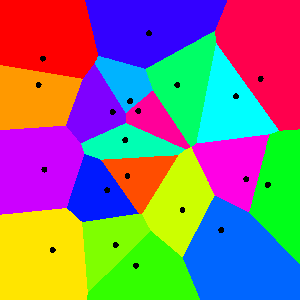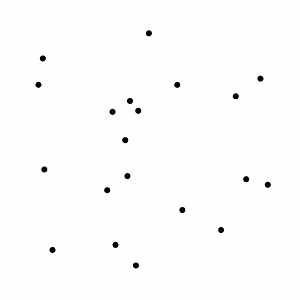# Voronoi Cells on a Plane

As a pilot of an (oversimplified) airplane, you may want to know how to communicate with the nearest control tower to receive information on local weather, air traffic, and so on. Each control tower has some region of the map that it “controls,” i.e., where it is closer than any other tower. An example is drawn below. These regions are known as the Voronoi regions (or Voronoi cells) of the points, and the whole diagram is the Voronoi decomposition determined by those points.The Voronoi diagram of 20 randomly chosen points in a square

For a given set of points, we can imagine the corresponding Voronoi regions as follows: grow disks around the points at the same rate, stopping when they hit each other. A disk collides with other disks exactly when its center ceases to be closest, so these regions are indeed the Voronoi cells.The Voronoi cells can be created by growing circles around the points.

Now let’s kick this into third gear—by which I mean the third dimension. In the animation above, suppose that the circles were moving downward over time, producing a cone below each point. By looking at these cones from above, we see exactly the Voronoi regions! Since cones are easy for computers to render, this provides a handy trick for quickly drawing Voronoi decompositions. In fact, this is how I drew all of these images!By placing identical, vertical cones under the points, one sees the corresponding Voronoi regions from directly above.

This construction is not only of theoretical value, but has also been put to good use packaging Ferrero Rocher chocolates:Voronoi regions in Ferrero Rocher packaging

Who knew these (delicious!) spherical candies were so mathematical?